# Governmental incentives for green bonds investment

## Abstract

Motivated by the recent studies on the green bond market, we build a Principal–Agent model in which an investor trades on a portfolio of green and conventional bonds, both issued by the same governmental entity. The government provides incentives to the bondholder in order to increase the amount invested in green bonds. These incentives are, optimally, indexed on the prices of the bonds, their quadratic variation and covariation. We show numerically on a set of French governmental bonds that our methodology outperforms the current tax-incentives systems in terms of green investments. Moreover, it is robust to model specification for bond prices and can be applied to a large portfolio of bonds using classical optimisation methods.

This is a preview of subscription content, access via your institution.

1. For a complete survey of renewable energy promotion policies, we refer to Table 3 in Della Croce, Kaminker, and Stewart .

2. The data provided below can be found at https://www.climatebonds.net/policy/policy-areas/tax-incentives.

3. See among others Mas-Colell, Whinston, and Green , Sannikov .

4. This literature has been growing since the study of the well-posedness of second-order backward stochastic differential equations, see for example Possamaï, Tan, and Zhou , or Soner, Touzi, and Zhang . A rigorous study of the Principal–Agent problem with volatility control in a general case can be found in Cvitanić, Possamaï, and Touzi .

5. We define the index as an average of the dynamics of the conventional bonds. In practice, the investor may trade a large quantity of conventional bonds and only a couple of green bonds. Thus, we argue that it is more convenient for the government to index the remuneration proposed on an average dynamics of conventional bonds in order to have more granularity for the green bonds’ incentives.

6. We force the control processes to be strictly positive so that the density of the canonical process in Appendix A is invertible and we can define properly the weak formulation of the control problem. Practically, this simply means that the investor ahs to invest in the index, and in at least one of the conventional and one of the green bonds.

7. See Appendix A for the weak formulation of the control problem, which explains how to construct $$\mathbb {P}^\pi$$.

8. We will see later that there might be several best responses of the Agent. Thus, following the tradition in the moral hazard literature, see Mas-Colell, Whinston, and Green , Sannikov  among others, we assume that the Principal has enough bargaining power to be able to choose the best response of the Agent that maximises his own utility.

9. The presented methodology can also be adapted to other choices of modelling.

10. We use the notation $$\mathbb {E}^{({\hat{\pi }}(t,Z_t,\Gamma _t))_{t\in [0,T]}}[\cdot ]=:\mathbb {E}^{{\hat{\pi }}(Z,\Gamma )}[\cdot ]$$

11. In practice, we observe that for the set of parameters we choose for the numerical experiences, the function $$h^{\text {obs}}$$ is strictly concave with respect to its last variable thus admits a unique maximizer $${\hat{\pi }}$$.

12. In particular, as the bond prices do not vary drastically during the year, we use 10 time steps, 40 space steps for the cash process, 10 for the stochastic rate and 20 for the risk factors of the green bond and index of conventional bonds.

13. Practically, we just choose a sufficiently large grid for values of c and perform optimization on the problem of the Agent to obtain the corresponding c. In this case, We obtain $$c=0.024$$.

## References

1. Agliardi, E., Agliardi, R.: Financing environmentally-sustainable projects with green bonds. Environ. Develop. Econ. 24(6), 608–623 (2019)

2. Aïd, R., Possamaï, D., Touzi, N.: Optimal electricity demand response contracting with responsiveness incentives. ArXiv preprintarXiv:1810.09063 (2018)

3. Global Sustainable Investment Alliance. 2016 global sustainable investment review. Technical report, GSIA (2017)

4. Ambec, S., Lanoie, P.: Does it pay to be green? A systematic overview. Acad. Manag Perspect. 22(4), 45–62 (2008)

5. Ang, A., Bhansali, V., Xing, Y.: Build America bonds. J. Fixed Income 20(1), 67–73 (2010)

6. International Capital Market Association. Green bond principles, 2016. Technical report, ICMA (2016)

7. Bachelet, M.J., Becchetti, L., Manfredonia, S.: The green bonds premium puzzle: the role of issuer characteristics and third-party verification. Sustainability 11(4), 1098 (2019)

8. Baker, M., Bergstresser, D., Serafeim, G., Wurgler, J.: Financing the response to climate change: the pricing and ownership of US green bonds. Technical Report w25194, National Bureau of Economic Research (2018)

9. Bauer, R., Smeets, P.: Social identification and investment decisions. J. Econ. Behav. Organ. 117, 121–134 (2015)

10. Bichteler, K.: Stochastic integration and $${L}^p$$-theory of semimartingales. Annals Probab. 9(1), 49–89 (1981)

11. Financial Stability Board. Global shadow banking monitoring report 2015. Technical report, FSB (2015)

12. Brooks, C., Oikonomou, I.: The effects of environmental, social and governance disclosures and performance on firm value: a review of the literature in accounting and finance. Br. Account. Rev. 50(1), 1–15 (2018)

13. Calabrese, T.D., Ely, T.L.: Borrowing for the public good: the growing importance of tax-exempt bonds for public charities. Nonprofit Volunt. Sect. Quarter. 45(3), 458–477 (2016)

14. Carr, P., Lee, R.: Robust replication of volatility derivatives. Mathematics in finance working paper series 2008–3, Courant Institute of Mathematical Sciences, New York University (2008)

15. Carr, P., Madan, D.: Option valuation using the fast Fourier transform. J. Comput. Finance 2(4), 61–73 (1999)

16. Chen, X., Sung, J.: Managerial compensation and outcome volatility. SSRN preprint 3140205 (2018)

17. Cvitanić, J., Possamaï, D., Touzi, N.: Moral hazard in dynamic risk management. Manag. Sci. 63(10), 3328–3346 (2017)

18. Cvitanić, J., Possamaï, D., Touzi, N.: Dynamic programming approach to principal-agent problems. Finance Stoch. 22(1), 1–37 (2018)

19. de Angelis, T., Tankov, P., Zerbib, O.D.: Environmental impact investing. SSRN preprint 3562534 (2020)

20. Della Croce, R., Kaminker, C., Stewart, F.: The role of pension funds in financing green growth initiatives. Working papers on finance, insurance and private pensions 10, OECD (2011)

21. Ekeland, I., Lefournier, J.: L’obligation verte : homéopathie ou incantation ? Technical report, Université Paris–Dauphine (2019)

22. Élie, R., Hubert, E., Mastrolia, T., Possamaï, D.: Mean-field moral hazard for optimal energy demand response management. Math. Finance 31(1), 399–473 (2021)

23. Febi, W., Schäfer, D., Stephan, A., Sun, C.: The impact of liquidity risk on the yield spread of green bonds. Finance Res. Lett. 27, 53–59 (2018)

24. Flammer, C.: Corporate green bonds. J. Financial Econ., to appear (2018)

25. Flammer, C.: Green bonds: effectiveness and implications for public policy. Environ. Energy Policy Econ. 1(1), 95–128 (2020)

26. Hachenberg, B., Schiereck, D.: Are green bonds priced differently from conventional bonds? J. Asset Manag. 19(6), 371–383 (2018)

27. Hernández Santibáñez, N., Mastrolia, T.: Contract theory in a VUCA world. SIAM J. Control Optim. 57(4), 3072–3100 (2019)

28. Hong, H., Kacperczyk, M.: The price of sin: the effects of social norms on markets. J. Financial Econ. 93(1), 15–36 (2009)

29. Klein, M.: Tax credit bonds. CitiBank Invest. Manag. Rev. 11, 27–31 (2009)

30. Krüger, P.: Corporate goodness and shareholder wealth. J. Financial Econ. 115(2), 304–329 (2015)

31. Leung, R.C.W.: Continuous-time principal–agent problem with drift and stochastic volatility control: with applications to delegated portfolio management. Technical report, Haas School of Business, University of California Berkeley (2014)

32. Lioui, A., Poncet, P.: Optimal benchmarking for active portfolio managers. Euro. J. Operat. Res. 226(2), 268–276 (2013)

33. Mas-Colell, A., Whinston, M.D., Green, J.: Microeconomic theory, vol. 1. Oxford University Press, New York (1995)

34. Mastrolia, T., Possamaï, D.: Moral hazard under ambiguity. J. Optim. Theory Appl. 179(2), 452–500 (2018)

35. Morel, R., Bordier, C.: Financing the transition to a green economy: their word is their (green) bond? Climate Brief 14 (2012)

36. Nilsson, J.: Investment with a conscience: examining the impact of pro-social attitudes and perceived financial performance on socially responsible investment behavior. J. Business Ethics 83(2), 307–325 (2008)

37. OECD: Mobilising the debt capital markets for a low carbon transition. Green finance and investment. Éditions OCDE, Paris (2017)

38. OECD: Investing in climate, investing in growth. Éditions OCDE, Paris (2017)

39. Ou-Yang, H.: Optimal contracts in a continuous-time delegated portfolio management problem. Rev. Financial Stud. 16(1), 173–208 (2003)

40. Park, S.K.: Investors as regulators: green bonds and the governance challenges of the sustainable finance revolution. Stanford J. Int. Law 54(1), 1–47 (2018)

41. Porter, M.E., Van der Linde, C.: Toward a new conception of the environment-competitiveness relationship. J. Econ. Perspect. 9(4), 97–118 (1995)

42. Possamaï, D., Tan, X., Zhou, C.: Stochastic control for a class of nonlinear kernels and applications. Annals Probab. 46(1), 551–603 (2018)

43. Sannikov, Y.: A continuous-time version of the principal-agent problem. Rev. Econ. Stud. 75(3), 957–984 (2008)

44. Schäl, M.: A selection theorem for optimization problems. Archiv der Mathematik 25(1), 219–224 (1974)

45. Soner, H.M., Touzi, N., Zhang, J.: Wellposedness of second order backward SDEs. Probability Theory and Related Fields 153(1–2), 149–190 (2012)

46. Soner, H.M., Touzi, N., Zhang, J.: Dual formulation of second order target problems. Annals Appl. Probab. 23(1), 308–347 (2013)

47. Sung, J.: Linearity with project selection and controllable diffusion rate in continuous-time principal-agent problems. RAND J. Econ. 26(4), 720–743 (1995)

48. Sung, J.: Optimal contracting under mean–volatility ambiguity uncertainties. SSRN preprint 2601174 (2015)

49. Tang, D.Y., Zhang, Y.: Do shareholders benefit from green bonds? J. Corp. Finance 61(101427), 1–18 (2020)

50. Zerbib, O.D.: The green bond premium. SSRN preprint 2890316 (2017)

51. Zerbib, O.D.: The effect of pro-environmental preferences on bond prices: evidence from green bonds. J. Bank. Finance 98, 39–60 (2019)

52. Zerbib, O.D.: A sustainable capital asset pricing model (S–CAPM): evidence from green investing and sin stock exclusion. SSRN preprint 3455090 (2019)

## Author information

Authors

### Corresponding author

Correspondence to Bastien Baldacci.

### Publisher's Note

Springer Nature remains neutral with regard to jurisdictional claims in published maps and institutional affiliations.

The datasets generated during and/or analysed during the current study are not publicly available due the fact that they are obtained through Bloomberg datasets, but are available from the corresponding author on reasonable request.

## Appendices

### Weak formulation of the problem

We work on the canonical space $$\mathcal {Q}$$ of continuous functions on [0, T] with Borel algebra $$\mathcal {F}$$. The $$(d^g+d^c+2)$$-dimensional canonical process is

\begin{aligned} \mathcal {B:}= \begin{pmatrix} X \\ W^g \\ W^c \\ W^I \end{pmatrix} \end{aligned}

and $$\mathbb {F}=(\mathcal {F}_t)_{t\in [0,T]}$$ is its natural filtration. We define $$\mathbb {P}_0$$ as the $$(d^g+d^c+1)$$-dimensional Wiener measure on $$\mathcal {Q}$$. Thus, $$\mathcal {B}$$ is a $$(d^g+d^c+2)$$-dimensional Brownian motion where $$(W^g,W^c,W^I)$$ has a correlation matrix $$\Sigma$$ under $$\mathbb {P}_0$$. We also define $$\mathcal {M}(\Omega )$$ as the set of probability measures on $$(\mathcal {Q},\mathcal {F}_T)$$ and

\begin{aligned} \mathbb {H}^2(\mathbb {P}_0)&:=\bigg \{(\pi _t)_{t\in [0,T]}:B\text { -valued}, \mathbb {F}\text {-predictable processes such that }\\&\qquad \mathbb {E}^{\mathbb {P}}\bigg [\int _0^T\Vert \pi _t\Vert _2^2 \mathrm {d}t \bigg ] <+\infty \bigg \}. \end{aligned}

We consider the following family of processes, indexed by $$\pi \in \mathbb {H}^2(\mathbb {P}_0)$$and define the set $$\mathcal {P}_m$$ as the set of probability measures $$\mathbb {P}^\pi \in \mathcal {M}(\mathcal {Q})$$ of the form

\begin{aligned} \mathbb {P}^\pi = \mathbb {P}_0 \circ (\mathcal {X}^\pi )^{-1}, \; \text {for all } \pi \in \mathbb {H}^2(\mathbb {P}_0). \end{aligned}

Thanks to Bichteler , we can define a pathwise version of the quadratic variation process $$\langle \mathcal {B}\rangle$$ and of its density process with respect to the Lebesgue measure $$\hat{\alpha }_t := \frac{d\langle \mathcal {B}\rangle _t}{\mathrm {d}t}$$. As the processes $$\pi \in \mathcal {A}\subset \mathbb {H}^2(\mathbb {P}_0)$$ have all their coordinates strictly positive, the volatility of $$\mathcal {B}$$ is invertible, which implies in particular that the process $$W_t = \int _0^t \hat{\alpha }_s^{-\frac{1}{2}}\mathrm {d}\mathcal {B}_s$$ is an $$\mathbb {R}^{d^g+d^c+2}$$-valued, $$\mathbb {P}$$–Brownian motion with correlation matrix $$\Sigma$$ for every $$\mathbb {P}\in \mathcal {P}_m$$. According to Soner, Touzi, and Zhang , there exists an $$\mathbb {F}^{\mathcal {B}}$$–progressively measurable mapping $$\beta _\pi : [0,T]\times \mathcal {Q} \longrightarrow \mathbb {R}^{d^g+d^c+2}$$ such that

\begin{aligned}&\mathcal {B} = \beta _\pi (\mathcal {X}^\pi ),\; \mathbb {P}_0\mathrm{--a.s}, \; W = \beta _\pi (\mathcal {B}),\; \mathbb {P}^\pi \mathrm{--a.s}, \\&\hat{\alpha }(\mathcal {B})= \pi \big (\beta _\pi (\mathcal {B})\big ), \; \mathrm {d}t \otimes \mathrm {d}\mathbb {P}^\pi \mathrm{--a.e.} \end{aligned}

In particular, the canonical process $$\mathcal {B}$$ admits the following dynamics for all $$\pi \in \mathcal {A}$$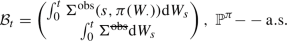The first coordinate of the canonical process is the desired output process, the $$d^g$$ next coordinates are the contractible sources of risk, that is the $$d^g$$ green bonds and the index of conventional bond, and the last $$d^c$$ coordinates are the non-contractible sources of risk. Then, we can introduce easily the drift of the output process by the means of Girsanov theorem. Denote

\begin{aligned} \frac{\mathrm {d}\mathbb {Q}}{\mathrm {d}\mathbb {P}^\pi } := \mathcal {E}\bigg (\int _0^\cdot {\tilde{\Sigma }}(s) \mathrm {d}W_s \bigg )_T, \end{aligned}

a change of measure independent of the control process $$\pi$$, where $$\tilde{\Sigma }: [0,T]\longrightarrow \mathcal {M}_{d^g+d^c+2}(\mathbb {R})$$ is such that

\begin{aligned} \tilde{\Sigma }(t) := \begin{pmatrix} \big (\frac{r^g(t)+\eta ^g(t)\circ \sigma ^g(t)}{\sigma ^g(t)}\big )^{\top } &{} \big (\frac{r^c(t)+\eta ^c(t)\circ \sigma ^c(t)}{\sigma ^c(t)}\big )^{\top } &{} \frac{\mu ^I(t)}{\sigma ^I(t)} \\ \mathbf {0}_{d^g+d^c+1,d^g} &{} \mathbf {0}_{d^g+d^c+1,d^c} &{}\mathbf {0}_{d^g+d^c+1,1} \end{pmatrix}. \end{aligned}

We finally obtain the desired dynamics for the output process and the $$d^g+d^c+1$$ sources of risk.

### We can define the functions Proof of Theorem 3.2

We can define the functions $$\sigma :[0,T]\times K \longrightarrow \mathcal {M}_{d^g+d^c+2,d^g+d^c+1}(\mathbb {R}), \lambda :[0,T]\longrightarrow \mathbb {R}^{d^g+d^c+1}$$ such that the set of contractible variables $$(B_t)_{t\in [0,T]}$$ can be rewritten for all $$\pi \in \mathcal {A}$$ as

\begin{aligned} \mathrm {d}B_t = \sigma (t,\pi _t) \big ( \lambda (t) \mathrm {d}t + \mathrm {d}W_t\big ), \end{aligned}
(B.1)

where for all $$(t,p)\in [0,T]\times K$$,

\begin{aligned}&\sigma (t,p) := \begin{pmatrix} \big (p^g \sigma ^g(t)\big )^\top &{} \big (p^c \sigma ^c(t)\big )^{\top } &{} p^I \sigma ^I(t) \\ \text {diag}(\sigma ^g(t)) &{} \mathbf {0}_{d^g,d^c} &{} \mathbf {0}_{d^g,1} \\ \mathbf {0}_{1,d^g}&{} \mathbf {0}_{1,d^c} &{} \sigma ^I(t) \\ \mathbf {0}_{d^c,d^g} &{} \text {diag}(\sigma ^c(t)) &{} \mathbf {0}_{d^c,1} \end{pmatrix},\; \\&\quad \lambda (t) := \begin{pmatrix} \big (\frac{r^g(t)+\eta ^g(t)\circ \sigma ^g(t)}{\sigma ^g(t)}\big )^{\top }&\big (\frac{r^c(t)+\eta ^c(t)\circ \sigma ^c(t)}{\sigma ^c(t)}\big )^{\top }&\frac{\mu ^I(t)}{\sigma ^I(t)} \end{pmatrix}^\top , \end{aligned}

Thanks to Assumption 2.2 and the definition of $$\mathcal {A}$$, the functions $$\sigma$$, and $$\lambda$$ are bounded. As the function $$\sigma (t,\pi )$$ is continuous in time for some constant control process $$\pi \in \mathcal {A}$$, there always exists a weak solution to (B.1). Thanks to the boundedness of the function $$\lambda$$, we can use Girsanov’s theorem which guarantees that every $$\pi \in \mathcal {A}$$ induces a weak solution for

\begin{aligned} B_t = B_0 + \int _0^t \sigma (s,\pi _s) \mathrm {d}W^{\prime }_s, \; \frac{\mathrm {d}\mathbb {P}^\prime }{\mathrm {d}\mathbb {P}}\bigg |_{\mathcal {F}_T} = \mathcal {E}\bigg (\int _0^\cdot \lambda (s)\cdot \mathrm {d}W_s \bigg )_T, \end{aligned}

where $$W^{\prime }$$ is a $$\mathbb {P}^{\prime }$$–Brownian motion.

The cost function $$k: K\rightarrow \mathbb {R}$$ is measurable and bounded by boundedness of the elements of K. We introduce the norms

\begin{aligned} \Vert Z^e\Vert _{\mathbb {H}^p}^p = \sup _{\pi \in \mathcal {A}}\mathbb {E}^{\pi }\bigg [\bigg ( \int _0^T\big \Vert \tilde{\sigma }(t,\pi _t) Z_t\big \Vert ^2 \mathrm {d}t\bigg )^{p/2}\bigg ], \; \Vert Y^e\Vert _{\mathbb {D}^p}^p = \sup _{\pi \in \mathcal {A}}\mathbb {E}^{\pi }\bigg [\sup _{t\in [0,T]} |Y_t|^p\bigg ], \end{aligned}

for any $$\mathbb {F}$$-predictable, $$\mathbb {R}^{d^g+d^c+2}$$-valued process $$Z^e$$ and $$\mathbb {R}$$-valued process $$Y^e$$, and for all $$(t,p)\in [0,T]\times K$$, $$\widetilde{\sigma }:[0,T]\times K\rightarrow \mathcal {M}_{d^g+d^c+2}(\mathbb {R})$$ is such that

\begin{aligned} \widetilde{\sigma }^2(t,\pi _t) = \sigma (t,p) \sigma ^\top (t,p). \end{aligned}

We also define the functions $$H^e: [0,T]\times \mathbb {R}^{d^g+d^c+2}\times \mathbb {S}_{d^g+d^c+2}(\mathbb {R})\times \mathbb {R}\longrightarrow \mathbb {R}$$ and $$h^e: [0,T]\times \mathbb {R}^{d^g+d^c+2}\times \mathbb {S}_{d^g+d^c+2}(\mathbb {R})\times \mathbb {R}\times K \longrightarrow \mathbb {R}$$ as

\begin{aligned}&H^e(t,z,g,y):=\sup _{p\in K}h^e(t,z,g,y,p), \\&h^e(t,z,g,y,p):= -\gamma k(p)y + z\cdot \sigma (t,p)\lambda (t) + \frac{1}{2}\mathrm {Tr}\Big [g\sigma (t,p)\Sigma (\sigma (t,p))^\top \Big ]. \end{aligned}

We introduce the set of so-called admissible incentives $$\mathcal {ZG}^e$$ as the set of $$\mathbb {F}$$-predictable processes $$(Z^e,\Gamma ^e)$$ valued in $$\mathbb {R}^{d^g+d^c+2}\times \mathbb {S}_{d^g+d^c+2}(\mathbb {R})$$ such that

\begin{aligned} \Vert Z^e\Vert _{\mathbb {H}^p}^p + \Vert Y^{e,Z^e,\Gamma ^e}\Vert _{\mathbb {D}^p}^p <+\infty , \end{aligned}
(B.2)

for some $$p>1$$ where for $$y^e_0\in \mathbb {R}$$,

\begin{aligned} Y_t^{e, y_0^e, Z^e,\Gamma ^e} := y_0^e + \int _0^t Z^e_s \mathrm {d}B_s + \frac{1}{2}\mathrm {Tr}\Big [\Gamma ^e_s \mathrm {d}\langle B\rangle _s \Big ] - H^e\big (s,Z_s^e,\Gamma _s^e,Y_s^{e, Z^e,\Gamma ^e}\big ) \mathrm {d}s. \end{aligned}

Condition (B.2) guarantees that the process $$(Y_t^{e, y_0^e, Z^e,\Gamma ^e})_{t\in [0,T]}$$ is well defined: provided that the right-hand side integrals are well defined, and by noting that $$H^e$$ is Lipschitz in its last variable (since the cost function k is bounded), $$(Y_t^{e, y_0^e, Z^e,\Gamma ^e})_{t\in [0,T]}$$ is the unique solution of an ODE with random coefficient. Moreover, as K is a compact set and $$h^e$$ is continuous with respect to its last variable, the supremum with respect to p is always attained. As $$(Z^e,\Gamma ^e)=(\mathbf {0}_{d^g+d^c+2},\mathbf {0}_{d^g+d^c+2,d^g+d^c+2})\in \mathcal {ZG}^e$$, this set is non-empty and we are in the setting of Cvitanić, Possamaï, and Touzi . Using [18, Proposition 3.3 and Theorem 3.6], we obtain that without reducing the utility of the Principal, any admissible contract admits the representation

\begin{aligned} U_A(\xi ) = Y_T^{e, Z^e,\Gamma ^e}, \end{aligned}

Define for all $$t\in [0,T]$$ the processes

\begin{aligned}&Z_t =: -\frac{Z_t^e}{\gamma Y_t^{e,y_0^e, Z^e,\Gamma ^e}}, \; \Gamma _t := -\frac{\Gamma _t^e}{\gamma Y_t^{e, y_0^e, Z^e,\Gamma ^e}},\; Y_t^{y_0,Z,\Gamma } \\&\quad = y_0 + \int _0^T Z_s \mathrm {d}B_s + \frac{1}{2}\mathrm {Tr}\big [\big (\Gamma _s + \gamma Z_s Z_s^\top \mathrm {d}\langle B\rangle _s \big ] - H\big (s,Z_s,\Gamma _s\big ) \mathrm {d}s, \end{aligned}

where $$H:[0,T]\times \mathbb {R}^{d^g+d^c+2}\times \mathbb {S}_{d^g+d^c+2}(\mathbb {R})\longrightarrow \mathbb {R}$$ is defined by $$H(t,z,g) = \sup _{p\in K} h(t,z,g,p)$$ and

\begin{aligned} \begin{aligned} \mathcal {ZG}:= \bigg \{(Z_t,\Gamma _t)_{t\in [0,T]}&: \mathbb {R}^{d^g+d^c+2}\times \mathbb {S}_{d^g+d^c+2}(\mathbb {R})\text {-valued, }\mathbb {F}\text {-predictable processes s.t}\\&\big (-\gamma Z_t U_A(Y_t^{y_0,Z,\Gamma }),-\gamma \Gamma _t U_A(Y_t^{y_0,Z,\Gamma })\big )_{t\in [0,T]}\in \mathcal {ZG}^e \bigg \}. \end{aligned} \end{aligned}
(B.3)

An application of Itō’s formula leads to $$\xi = Y_T^{y_0,Z,\Gamma }$$. Thus, we obtain the desired representation for admissible contracts and $$V_A( Y_T^{y_0,Z,\Gamma })=U_A(y_0)$$. The characterisation of $$\mathcal {A}(Y_T^{y_0,Z,\Gamma })$$ is a direct consequence of Cvitanić, Possamaï, and Touzi [18, Proposition 3.3].

### Framework

In the article, we considered a deterministic structure for the short-term rates. However, this omits some important stylised facts of the yield curve. In this section we show that at the expense of the use of stochastic control, the government can provide incentives based on short-term rates following a one factor stochastic model.

We now assume that the vectors of short rate dynamics of the green bonds are given by

\begin{aligned} \mathrm {d}r_t^{g} := a^{g}(t,r_t^g) \mathrm {d}t + \text {diag}(b^{g}) \mathrm {d}W_t^{g,r}, \end{aligned}
(C.1)

where $$b^g\in \mathbb {R}^{d^g}_+$$, $$a^{g}:[0,T]\times \mathbb {R}^{d^g}\longrightarrow \mathbb {R}^{d^g}$$ and $$W^{g,r}$$ is a $$d^g$$-dimensional Brownian motion of correlation matrix $$\Sigma ^{g,r}$$.

### Remark C.1

For notational simplicity, we assume no dependence between the risk sources of the short-term rates and the ones of the bonds. Allowing such dependence is straightforward and does not lead to a higher dimension of the control problem.

We contract only on the portfolio process, the risk factors of the green bonds and of the stochastic short-term rate of the green bonds, and the risk factor of the index of conventional bonds. The new sets of state variables arewhere the superscript S stands for stochastic, which can be written aswhere for all $$t\in [0,T]$$, $$p=(p^g,p^c,p^I)\in \mathbb {R}^{d^g}\times \mathbb {R}^{d^c}\times \mathbb {R}$$, $$r^g\in \mathbb {R}^{d^g}$$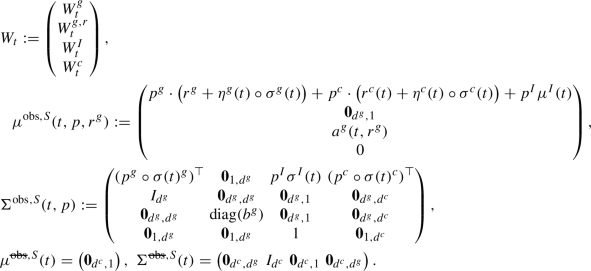We now specify the new set of admissible contracts that we consider for the incentives proposed by the government.

Define $$\mathcal {C}^S$$ as the set of admissible contracts in the case of stochastic short-term rates (the admissibility conditions are the same as for the set $$\mathcal {C}$$) and for any $$\pi \in \mathcal {A}$$ we introduce the following quantities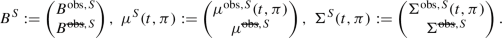We define $$h^S:[0,T]\times \mathbb {R}^{2d^g+d^c+2}\times \mathbb {S}_{2d^g+d^c+2}(\mathbb {R})\times \mathbb {R}^{d^g}\times K\longrightarrow \mathbb {R}$$ such that

\begin{aligned} h^S(t,z,g,r^g,p) = -k(p) + z \cdot \mu ^S(t,p,r^g) + \frac{1}{2}\mathrm {Tr}\Big [ g\Sigma ^S(t,p)\Sigma (\Sigma ^S(t,p))^{\top } \Big ], \end{aligned}

and for all $$(t,z,g,r^g)\in [0,T]\times \mathbb {R}^{2d^g+d^c+2}\times \mathbb {S}_{2d^g+d^c+2}(\mathbb {R})$$ we define

\begin{aligned} \mathcal {O}^S(t,z,g,r^g):= \Big \{{\hat{p}}\in K: {\hat{p}} \in \underset{p\in K}{\text {argmax }}h^S(t,z,g,r^g,p)\Big \}, \end{aligned}

as the set of maximisers of $$h^S$$ with respect to its last variable for $$(t,z,g,r^g)$$ fixed. Following Schäl , there exists at least one Borel-measurable map $${\hat{\pi }}:[0,T]\times \mathbb {R}^{2d^g+d^c+2}\times \mathbb {S}_{2d^g+d^c+2}(\mathbb {R})\times \mathbb {R}^{d^g}\longrightarrow K$$ such that for every $$(t,z,g,r^g)\in [0,T]\times \mathbb {R}^{2d^g+d^c+2}\times \mathbb {S}_{2d^g+d^c+2}(\mathbb {R})\times \mathbb {R}^{d^g}$$, $${\hat{\pi }}(t,z,g,r^g)\in \mathcal {O}^S(t,z,g,r^g)$$. We denote by $$\mathcal {O}^S$$ the set of all such maps. By analogy with Theorem 3.2, the following theorem states the form of any admissible contracts in this setting.

### Theorem C.2

Without reducing the utility of the Principal, we can restrict the study of admissible contracts to the set $$\mathcal {C}^S_1$$ where any $$\xi \in \mathcal {C}^S_1$$ is of the form $$\xi =Y_T^{y_0,Z^S,\Gamma ^S,{\hat{\pi }}}$$ where for all $$t\in [0,T]$$,

\begin{aligned} Y_t^{y_0,Z^S,\Gamma ^S,{\hat{\pi }}}&:= y_0 + \int _0^t Z^S_s\cdot \mathrm {d}B_s + \frac{1}{2}\mathrm {Tr}\Big [\big (\Gamma ^S_s + \gamma Z^S_s (Z_s^S)^{\top }\big )\mathrm {d}\langle B^S\rangle _s\Big ]\nonumber \\&\quad - h^S\big (s,Z^S_s,\Gamma ^S_s,r_s^g,{\hat{\pi }}(s,Z_s,\Gamma _s,r_s^g)\big )\mathrm {d}s, \end{aligned}
(C.2)

where $${\hat{\pi }}\in \mathcal {O}^S$$ and $$(Z^S_t)_{t\in [0,T]}, (\Gamma _t^S)_{t\in [0,T]}$$ are respectively $$\mathbb {R}^{2d^g+2+d^c}$$ and $$\mathbb {S}_{2d^g+2+d^c}(\mathbb {R})$$-valued, $$\mathbb {F}$$-predictable processes satisfying similar conditions as the elements of $$\mathcal {ZG}$$. We denote the set of admissible incentives as $$\mathcal {ZG}^S$$. Moreover in the present case of stochastic rates for green bonds

\begin{aligned}&V^A(Y_T^{y_0,Z^S,\Gamma ^S,{\hat{\pi }}})=U_A(y_0),\;\\&\quad \mathcal {A}\big (Y_T^{y_0,Z^S,\Gamma ^S,{\hat{\pi }}}\big ) = \Big \{\big ({\hat{\pi }}(t,Z^S_t,\Gamma ^S_t,r_t^g)\big )_{t\in [0,T]}, \hat{\pi }\in \mathcal {O}^S, (Z^S_t,\Gamma ^S_t)_{t\in [0,T]}\in \mathcal {ZG}^S\Big \}. \end{aligned}

We now set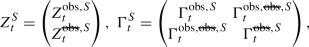where for all $$t\in [0,T]$$We define $$h^{\text {obs},S}:[0,T]\times \mathbb {R}^{2d^g+2}\times \mathbb {S}_{2d^g+2}(\mathbb {R})\times \mathbb {R}^{d^g}\times K\longrightarrow \mathbb {R}$$ such that

\begin{aligned}&h^{\text {obs}}(t,z^{\text {obs},S},g^{\text {obs},S},r^g,p) = -k(p) + z^{\mathrm{obs},S} \cdot \mu ^{\text {obs},S}(t,p,r^g)\\&\quad + \frac{1}{2}\mathrm {Tr}\Big [ g^{\mathrm{obs},S}\Sigma ^{\mathrm{obs},S}(t,p)\Sigma (\Sigma ^{\mathrm{obs},S}(t,p))^{\top } \Big ], \end{aligned}

and for all $$(t,z^{\text {obs},S},g^{\text {obs},S},r^g)\in [0,T]\times \mathbb {R}^{2d^g+2}\times \mathbb {S}_{2d^g+2}(\mathbb {R})\times \mathbb {R}^{d^g}$$ we define

\begin{aligned} \mathcal {O}^{\text {obs},S}(t,z^{\text {obs}},g^{\text {obs}},r^g) := \Big \{{\hat{p}}\in K: {\hat{p}}\in \underset{p\in K}{\text {argmax }}h^{\text {obs},S}(t,z^{\text {obs},S},g^{\text {obs},S},r^g,p)\Big \}. \end{aligned}

Using again Schäl , there exists one Borel-measurable map $${\hat{\pi }}:[0,T]\times \mathbb {R}^{2d^g+2}\times \mathbb {S}_{2d^g+2}(\mathbb {R})\times \mathbb {R}^{d^g}\longrightarrow ~B$$ such that for every $$(t,z^{\text {obs},S},g^{\text {obs},S},r^g)\in [0,T]\times \mathbb {R}^{2d^g+2}\times \mathbb {S}_{2d^g+2}(\mathbb {R})\times \mathbb {R}^{d^g}$$, $${\hat{\pi }}(t,z^{\text {obs},S},g^{\text {obs},S},r^g)\in \mathcal {O}^{\text {obs},S}(t,z^{\text {obs},S},g^{\text {obs},S},r^g)$$ and $$\mathcal {O}^{\text {obs},S}$$ denotes the set of all such maps. We consider the subset of admissible contracts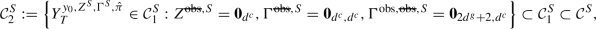where any contract in $$\mathcal {C}_2^S$$ is of the form $$Y_T^{y_0,Z^{\text {obs},S},\Gamma ^{\text {obs},S},{\hat{\pi }}}$$ where for all $$t\in [0,T]$$,

\begin{aligned} \begin{aligned} Y_t^{y_0,Z^{\text {obs},S},\Gamma ^{\text {obs},S},{\hat{\pi }}} := y_0 + \int _0^t&Z_s^{\mathrm{obs},S}\cdot \mathrm {d}B_s^{\mathrm{obs},S} + \frac{1}{2}\mathrm {Tr}\Big [\big (\Gamma ^{\mathrm{obs},S}_s + \gamma Z_s^{\mathrm{obs},S}(Z_s^{\mathrm{obs},S})^{\top }\big )d\langle B^{\mathrm{obs},S}\rangle _s\Big ] \\&- h^{\mathrm{obs},S}\Big (s,Z_s^{\mathrm{obs}},\Gamma _s^{\mathrm{obs}},r_s^g,{\hat{\pi }}\big (s,Z_s^{\mathrm{obs},S},\Gamma _s^{\mathrm{obs},S},r_s^g\big )\Big )\mathrm {d}s, \end{aligned} \end{aligned}
(C.3)

where $$y_0\ge 0$$, $${\hat{\pi }}\in \mathcal {O}^{\text {obs},S}$$ and $$(Z^{\text {obs},S},\Gamma ^{\text {obs},S})\in \mathcal {ZG}^{\text {obs},S}$$ with

\begin{aligned} \mathcal {ZG}^{\text {obs},S}&:= \Big \{(Z^{\text {obs},S},\Gamma ^{\text {obs},S}): \mathbb {R}^{2d^g+2}\times \mathbb {S}_{2d^g+2}(\mathbb {R})\text {-valued, }\\&\quad \mathbb {F}\text {-predictable s.t } Y_T^{y_0,Z^{\text {obs},S},\Gamma ^{\text {obs},S},{\hat{\pi }}}\in \mathcal {C}_2^S \Big \}. \end{aligned}

We can now formulate the stochastic control problem faced by the government.

### The Hamilton–Jacobi–Bellman equation

Let us define the process $$(Q^{y_0,Z^{\text {obs},S},\Gamma ^{\text {obs},S},{\hat{\pi }}}_t)_{t\in [0,T]}$$ where for all $$(t,y_0,Z^{\text {obs},S},\Gamma ^{\text {obs},S},{\hat{\pi }})\in [0,T]\times \mathbb {R}\times \mathcal {ZG}^{\text {obs},S}\times \mathcal {O}^{\text {obs},S}$$

\begin{aligned} Q_t^{y_0,Z^{\text {obs},S},\Gamma ^{\text {obs},S},{\hat{\pi }}}&:= X_t - \int _0^t \sum _{i=1}^{d^g}\big (G_i -{\hat{\pi }}_i^{g}(s,Z_s^{\mathrm{obs},S},\Gamma _s^{\mathrm{obs},S},r_s^g)\big )^2 ds -Y_t^{y_0,Z^{\text {obs},S},\Gamma ^{\text {obs},S},{\hat{\pi }}}. \end{aligned}

The optimisation problem of the government that we consider here is

\begin{aligned} \begin{aligned} \widetilde{V}_0^P = \sup _{y_0\ge 0}\sup _{(Z^{\text {obs},S},\Gamma ^{\text {obs},S},{\hat{\pi }})\in \mathcal {ZG}^{\text {obs},S}\times \mathcal {O}^{\text {obs},S}}\mathbb {E}^{{\hat{\pi }}(Z^{\text {obs},S},\Gamma ^{\text {obs},S})}&\Bigg [-\exp \Big (-\nu Q_T^{y_0,Z^{\text {obs},S},\Gamma ^{\text {obs},S},{\hat{\pi }}}\Big )\Bigg ]. \end{aligned} \end{aligned}
(C.4)

Due to the presence of state variables in the best response of the Agent, the optimal control of the Principal will no longer be deterministic, and we have to rely on the Hamilton–Jacobi–Bellman formulation of the stochastic control problem. First, we note that the supremum over $$y_0$$ is attained by setting $$y_0=0$$. Next, the state variables of the control problem are $$\big (t,B_t^{\text {obs},S},Q_t^{0,Z^{\text {obs},S},\Gamma ^{\text {obs},S},{\hat{\pi }}}\big )$$ and as it is standard in control problems with CARA utility function, the last variable can be simplified. Define $$P^S=\mathbb {R}^{2d^g+2}\times \mathbb {S}_{2d^g+2}(\mathbb {R})$$, and the Hamiltonian $$H^{{\hat{\pi }}}:[0,T]\times P^S \times \mathbb {R}\times P^S \longrightarrow \mathbb {R}$$

\begin{aligned}&H^{\hat{\pi }}(t,z,g,u,u_b,u_{bb}) := \nu u \bigg ( z \cdot \mu ^{\mathrm{obs},S}\big (t,{\hat{\pi }}(t,z,g,r^g),r^g\big ) + \sum _{i=1}^{d^g}\big (G_i -{\hat{\pi }}_i^{g}(t,z,g,r^g)\big )^2 \\&+ \frac{1}{2}\mathrm {Tr}\bigg [(g+\gamma z z^{\top })\Sigma ^{\mathrm{obs},S}\big (t,{\hat{\pi }}(t,z,g,r^g)\big )\Big (\Sigma ^{\mathrm{obs},S}\big (t,{\hat{\pi }}(t,z,g,r^g)\big )\Big )^{\top }\bigg ] \\&\quad - h^{\mathrm{obs},S}\big (t,z,g,r^g,{\hat{\pi }}(t,z,g,r^g)\big ) \bigg ) \\&+ \frac{1}{2} \nu ^2 u \mathrm {Tr}\bigg [z z^{\top } \Sigma ^{\mathrm{obs},S}\big (t,{\hat{\pi }}(t,z,g,r^g)\big )\Big (\Sigma ^{\mathrm{obs},S}\big (t,{\hat{\pi }}(t,z,g,r^g)\big )\Big )^{\top }\bigg ] \\&\quad + u_b \cdot \mu ^{\mathrm{obs},S}\big (t,{\hat{\pi }}(t,z,g,r^g),r^g\big ) \\&+ \frac{1}{2}\mathrm {Tr}\bigg [ \Sigma ^{\mathrm{obs},S}\big (t,{\hat{\pi }}(t,z,g,r^g)\big )\Big (\Sigma ^{\mathrm{obs},S}\big (t,{\hat{\pi }}(t,z,g,r^g)\big )\Big )^{\top } u_{bb} \bigg ]. \end{aligned}

The value function of the control problem of the Principal is related to the following Hamilton–Jacobi–Bellman equation

\begin{aligned} \begin{aligned} {\left\{ \begin{array}{ll} \partial _t U(t,b) + \underset{(z,g,{\hat{\pi }})\in P^S \times \mathcal {O}^{\text {obs},S}}{\sup } H^{\hat{\pi }}\big (t,z,g,b,U,U_b,U_{bb}\big ) =0,\\ U(T,b)= -1, \end{array}\right. } \end{aligned} \end{aligned}
(C.5)

where $$U:[0,T]\times \mathbb {R}^{2d^g+2}\longrightarrow \mathbb {R}$$ and for all $$(i,j)\in \{1,\dots ,2d^g+2\}$$, $$(U_b)_i = \partial _{b_i} U, (U_{bb})_{i,j}=\partial _{b_i b_j}U$$, in the sense that $${\widetilde{V}}^P_0=U(0,b_0)$$ where $$B_0^{\text {obs},S}=b_0$$ and $$y_0=0$$. Thus, the incentives provided to the investor are obtained up to the resolution of a $$(2d^g + 2)$$-dimensional HJB equation. Although it provides greater flexibility on the modelling of short-term rates, this approach can only be applied to a small portfolio of bonds using classic numerical schemes on sparse grids.

## Rights and permissions

Reprints and Permissions